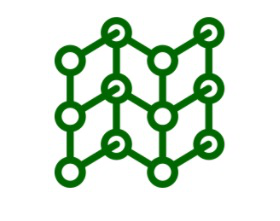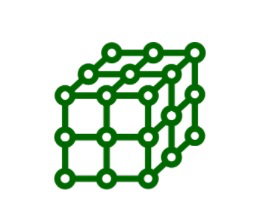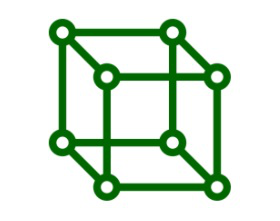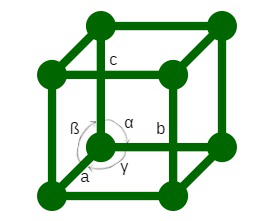GFG App
Open AppBrowser
Continue

In crystalline solids, their constituent particles have a definite arrangement in three dimensions. The positions of these particles in the crystal relative to each other are usually represented by points. The dispensation of these unendurable sets of points is called a space lattice. The positions occupied by atoms, ions, or molecules in a crystal lattice are the collar lattice points or lattice sites. This article will cover the basic understanding of space lattices, two-dimension lattices, and then three-dimensional lattices. Let’s start one by one.

### Two Dimensional Lattices

A two-dimensional lattice is a stationary distribution of constituent particles (atoms, ions, or molecules) in the plane. There are five types of two-dimensional lattices. These are square rectangles, parallelograms, rhombi, and hexagonal lattices. These differ in the symmetry of the arrangement of the points. The hexagonal lattice has the most symmetrical arrangement of points while the parallelogram lattice has the least symmetric arrangement of points.Two Dimensional Lattices

Because of the regularly repeating arrangement of points in a two-dimensional lattice, a small portion of the lattice needs to be described to fully specify it. For example, select four points in a two-dimensional lattice and join them to form a parallelogram. This smallest part is called the unit cell. An ideal lattice can be produced by repeatedly rotating the unit cell in the direction of its edges by a distance equal to the edge of the cell.

A unit cell gives the shape of the entire lattice. However, it can be noted that for any given lattice, unit cells can be selected in many different ways. This is because a lattice contains a very large number of atoms and many identical points can be found.

A cell with an interior point is called a focused unit cell. Those unit cells which do not have any internal point are called primitive unit cells.

Thus, a two-dimensional lattice can be described as specifying the unit cell by the lengths of the edges and the angles between them. Thus, the five two-dimensional lattices are:

1. Square lattice
2. Rectangular lattice
3. Parallelogram lattice
4. Rhombic Lattice
5. Hexagonal Lattice

### Three Dimensional Crystal Lattice

The constituent particles (atoms, ions, or molecules) in a crystalline solid have a definite three-dimensional arrangement. If the three-dimensional arrangement of the constituent particles in a crystal is represented diagrammatically by depicting each particle by points, the arrangement is called a crystal lattice or space lattice. A crystal lattice is a well-ordered arrangement of the constituent particles of a crystalline solid in three-dimensional space.Three Dimensional Crystal Lattice

A three-dimensional crystal lattice is like a two-dimensional lattice. The set of repeated lattice points in a crystal lattice can be found by carefully analyzing the crystal lattice This undershot Ingeminate pattern is called a unit cell. A unit cell can be defined as the smallest three-dimensional repetitive part of the space lattice, which when repeated in different directions produces a complete crystal lattice.Unit Cell

This smallest Ingeminate pattern represents the size of the entire crystal. A complete lattice can be produced by repeatedly rotating the unit cell in the direction of its edges from a distance equal to the edge of the cell. Crystals can be thought of as consisting of an infinite number of unit cells.

## Crystal Lattice

A regular three-dimensional arrangement of points (ions, atoms, etc.) in space is called a crystal lattice. There are 14 possible three-dimensional lattices. These are called Bravais lattices.

Characteristics of Crystal Lattice

• Each point in the crystal lattice is called a lattice point or lattice site.
• Each point in the crystal lattice represents a constituent particle which can be an atom, a molecule (a group of atoms) or an ion.
• Three-dimensional distribution of lattice points representing a crystal lattice.
• The lattice points are joined by straight lines to bring out the geometry of the lattice.

## Unit Cell

The unit cell is the smallest part of the crystal lattice, which when repeated in different directions produces the entire lattice, e.g. Primitive unit cells and concentrated unit cells.

Types of Unit Cells: Unit cells can be broadly divided into two categories,

• Primitive unit cell – In primitive unit cells, the constituent particles are present only at the corner positions of the unit cell whereas, in concentrated unit cells, one or more component particles are present at locations other than the corners.
• Centered unit cell  Centered unit cell is classified as the body-centered unit cell (bcc), face-centered unit cell (fcc), end centered unit cell (ECC).

Parameters of a Unit CellA unit cell is characterized by:

• Its dimensions (length) are of form a, b, and c with three edges. These edges may or may not be mutually perpendicular.
• angles α, ß, and γ between the pair of edges. The angle α is between the edges b and c, angle ß is between the edges c and a and angle γ is between the edges a and b. Thus, a unit cell is characterized by six parameters, a, b, c, α, ß and γ. The complete crystal lattice can be obtained by expanding the unit cell in all three directions.

## Sample Questions

Question 1: What are the characteristics of this unit cell?

The most convenient cell is the smallest unit cell that has perfect lattice symmetry.

• For square, rectangular, and parallelogram lattice, the unit cells chosen are square, rectangle, and parallelogram respectively.
• For a hexagonal lattice, the unit cell is a rhombus with an angle of 60°.
• For a rhombus lattice, a rectangular unit cell with one interior point is usually chosen.

Question 2: Which network solid is an exceptionally good conductor of electricity?

Graphite, a network solid, is a good conductor of electricity.

Question 3. How are unit cell and space lattice related?

The space lattice is obtained by repeating the unit cell in three dimensions. The spatial arrangement, stump, and density of the unit cell and space lattice are equivalent.

Question 3: Pick out the odd ones from the following sets: Sulphur, Argon, Solid Co2, Diamond, SIC, Quartz, BaO, Graphite

Diamond because all other molecular solids and BaO because all are covalent solids.

Question 4: What is the difference between glass and quartz, while both are made of SiO4, tetrahedral? Under what conditions can quartz be converted to glass?